Question

2. Consider the function f : R2 → R defined below. r3уг_ if (x,y) (0,0) f(x,y)...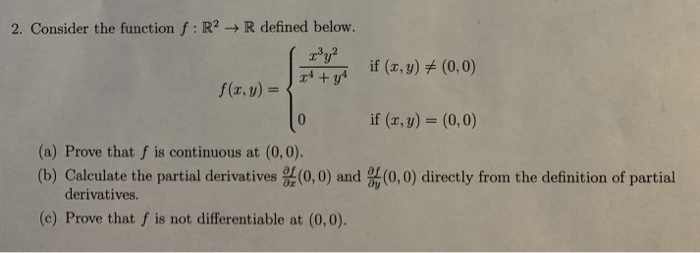2. Consider the function f : R2 → R defined below. r3уг_ if (x,y) (0,0) f(x,y) = if (x, y) (0, 0) (a) Prove that f is continuous at (0,0) (b) Calculate the partial derivatives (0,0) and (0,0) directly from the definition of partial derivatives. (c) Prove that f is not differentiable at (0,0).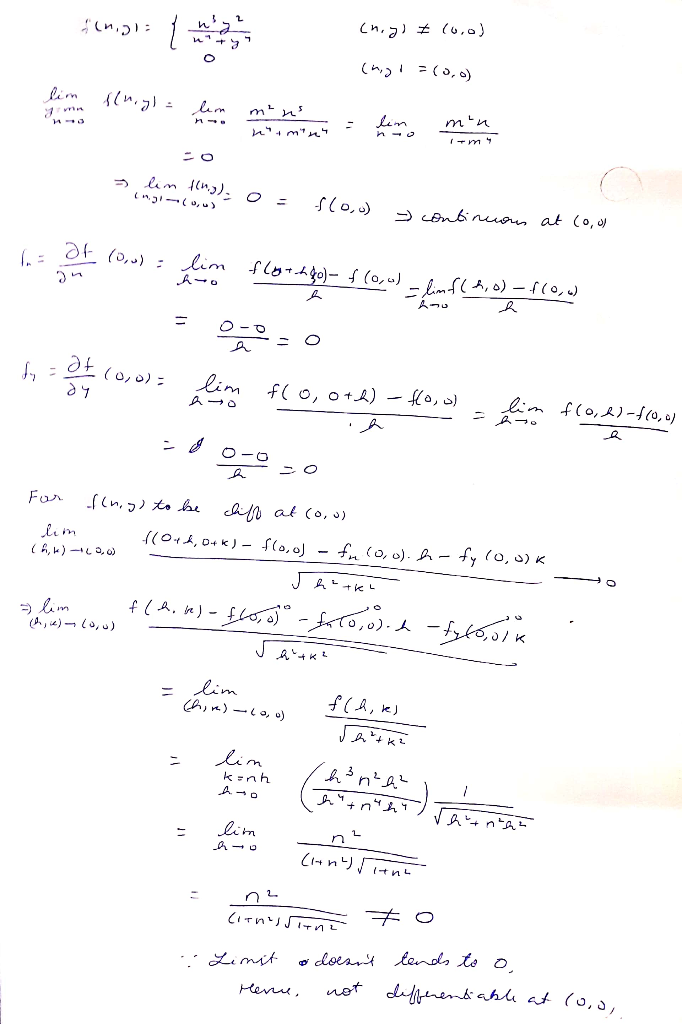Earn Coins

Coins can be redeemed for fabulous gifts.

Similar Homework Help Questions
• if (r.y) (0,0), 0,f (, y) (0, 0) 2. Consider f : IR2 -R defined by f(r,y)-+ (a) Show by explicit computation that the directional derivative exists at (x, y)- (0,0) for all oi rections u є R2 with 1...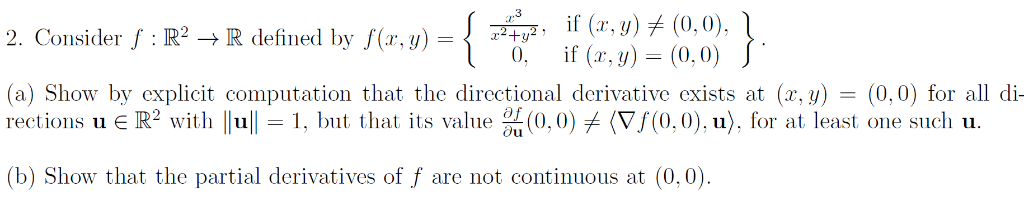if (r.y) (0,0), 0,f (, y) (0, 0) 2. Consider f : IR2 -R defined by f(r,y)-+ (a) Show by explicit computation that the directional derivative exists at (x, y)- (0,0) for all oi rections u є R2 with 1 11-1, but that its value %(0.0) (Vf(0,0).u), fr at least one sucli u. (b) Show that the partial derivatives of f are not continuous at (0,0) if (r.y) (0,0), 0,f (, y) (0, 0) 2. Consider f : IR2 -R...

• = f ху Consider the function f(x,y) if x² + y² (x, y) + (0,0) =...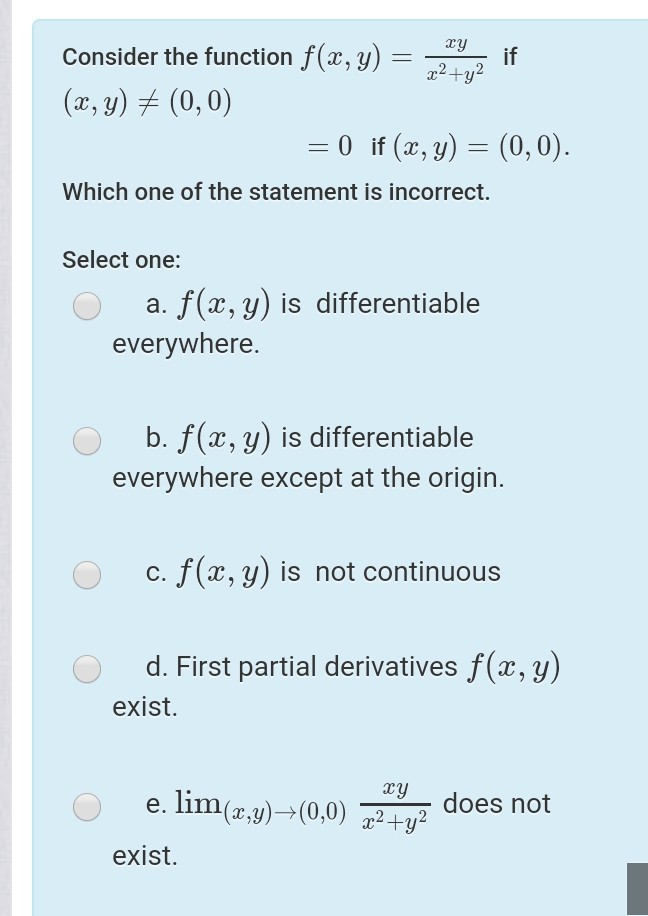= f ху Consider the function f(x,y) if x² + y² (x, y) + (0,0) = 0 if (x, y) = (0,0). Which one of the statement is incorrect. Select one: a. f(x,y) is differentiable everywhere. b. f(x,y) is differentiable everywhere except at the origin. c. f(x,y) is not continuous d. First partial derivatives f(x,y) exist. ху e. lim(x,y)—(0,0) x2 + y2 does not exist.

• *Let f : R2 -R be given by z, y)(0,0 r, y)- 2y and f(0,0) =...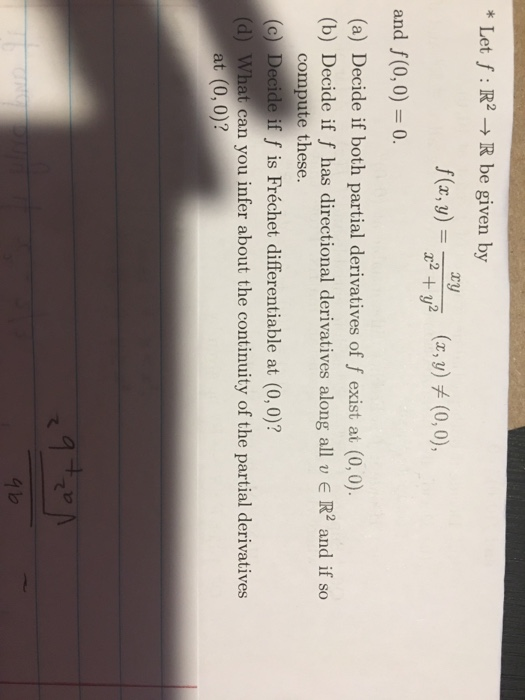*Let f : R2 -R be given by z, y)(0,0 r, y)- 2y and f(0,0) = 0. (a) Decide if both partial derivatives of f exist at (0, 0) (b) Decide if f has directional derivatives along all v R2 and if so compute these. (c) Decide if f is Fréchet differentiable at (0, 0)? (d) What can you infer about the continuity of the partial derivatives at (0, 0)? て

• Question 2 (20 points): Consider the functions f(x, y)-xe y sin y and g(x, y)-ys 1. Show f is differentiable in its domain 2. Compute the partial derivatives of g at (0,0) 3. Show that g is not d...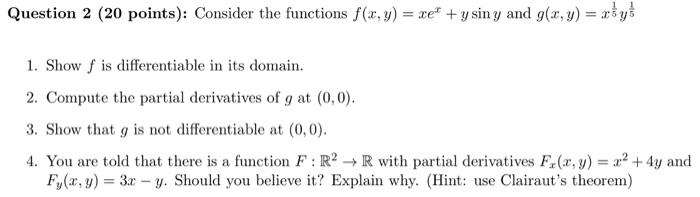Question 2 (20 points): Consider the functions f(x, y)-xe y sin y and g(x, y)-ys 1. Show f is differentiable in its domain 2. Compute the partial derivatives of g at (0,0) 3. Show that g is not differentiable at (0,0) 4. You are told that there is a function F : R2 → R with partial derivatives F(x,y) = x2 +4y and Fy(x, y 3x - y. Should you believe it? Explain why. (Hint: use Clairaut's theorem) Question 2...

• Exercice 2 (5pts) Let f given by f(x, y) Isinyif (x, y) (0,0) and f(0,0) 0...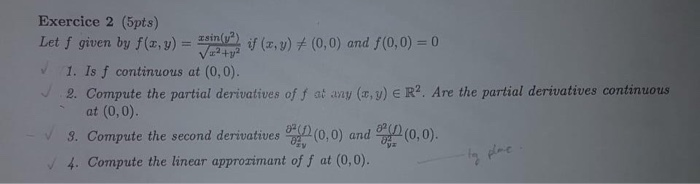Exercice 2 (5pts) Let f given by f(x, y) Isinyif (x, y) (0,0) and f(0,0) 0 1V224 1. Is f continuous at (0,0). 2. Compute the partial derivatives of f at any (x, y) E R2. Are the partial derivatives continuous (0,0). at (0,0) (0,0) and 3. Compute the second derivatives 4. Compute the linear approzimant of f at (0,0). Exercice 2 (5pts) Let f given by f(x, y) Isinyif (x, y) (0,0) and f(0,0) 0 1V224 1. Is f...

• (1 point) Consider the function defined by F(x, y) = x2 + y2 except at (r, y) - (0, 0) where F(0,0)0 Then we have (0,0)...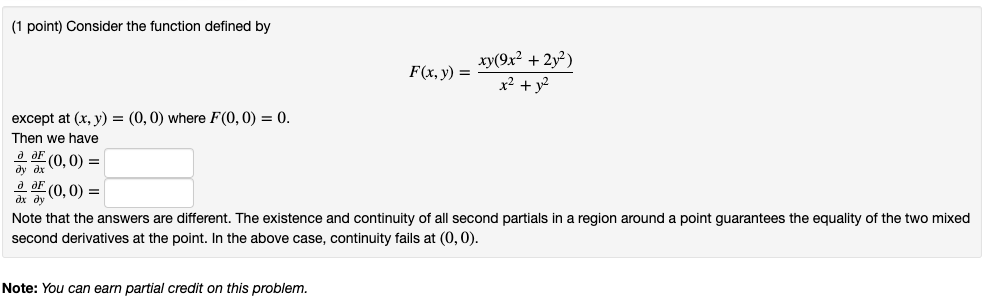(1 point) Consider the function defined by F(x, y) = x2 + y2 except at (r, y) - (0, 0) where F(0,0)0 Then we have (0,0) = (0,0) = ax dy Note that the answers are different. The existence and continuity of all second partials in a region around a point guarantees the equality of the two mixed second derivatives at the point. In the above case, continuity fails at (0,0) Note: You can earn partial credit on this problem...

• the function of two real variables defined below: 1 –9x + 2y“ (x, y) + (0,0),...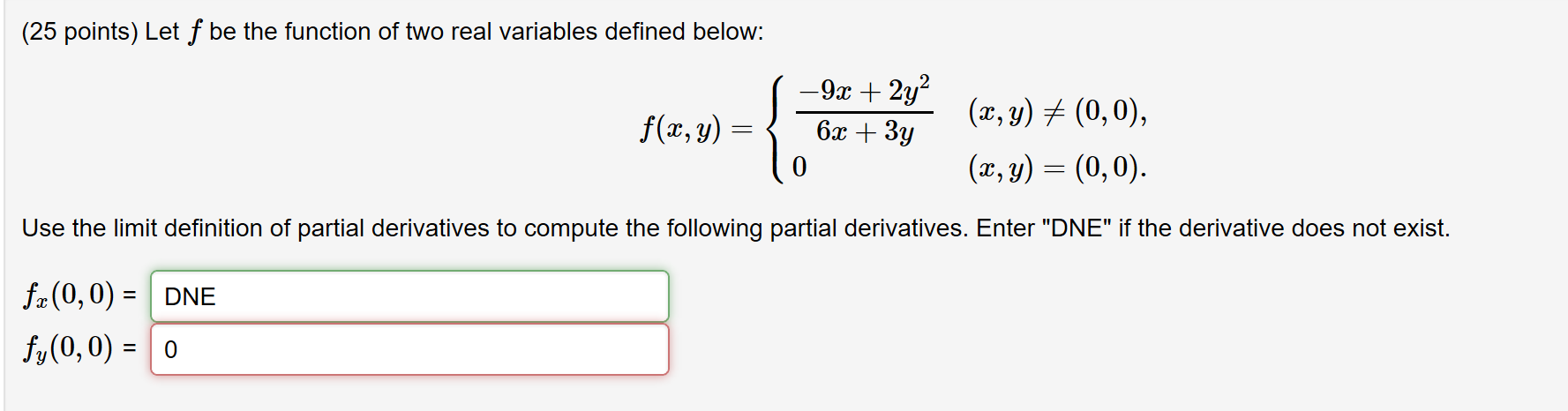the function of two real variables defined below: 1 –9x + 2y“ (x, y) + (0,0), f(x, y) = { 6x + 3y 10 (x, y) = (0,0). Use the limit definition of partial derivatives to compute the following partial derivatives. Enter "DNE" if the derivative does not exist. fx(0,0) = DNE fy(0,0) = 0

• Please answer fully and clearly. Thank you. )if (e-) (0.0)) if (z, y)#10, 0), 2. Consider f R2R defined by f(,) plHP, 0, if (x,y) (0,0)J (a) Show by explicit computation that the directional derivati...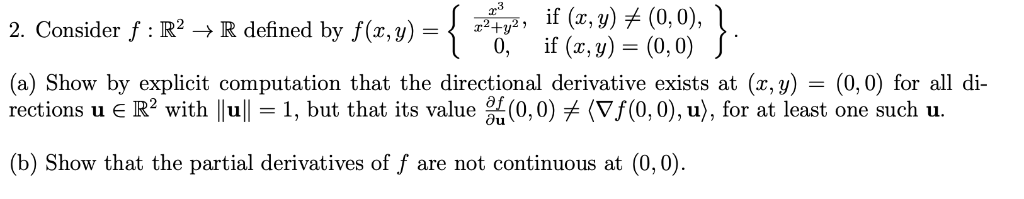Please answer fully and clearly. Thank you. )if (e-) (0.0)) if (z, y)#10, 0), 2. Consider f R2R defined by f(,) plHP, 0, if (x,y) (0,0)J (a) Show by explicit computation that the directional derivative exists at (x, y) - (0,0) for all di rections u R2 with lull-1, but that its value (0,0)メ(Vf(0,0), u), for at least one such u. b) Show that the partial derivatives of f are not continuous at (0,0) )if (e-) (0.0)) if (z, y)#10,...

• 2. [1 mark] Calculate the limit of the vector valued function f: ACRY-R lim G logy)...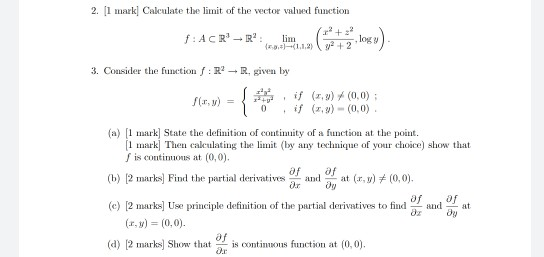2. [1 mark] Calculate the limit of the vector valued function f: ACRY-R lim G logy) 3. Consider the function :R? - R. given by Flv = 0 if if (,y) (0,0): (x,y) -(0,0) (a) (1 mark] State the definition of continuity of a function at the point. (1 mark] Then calculating the limit (by any technique of your choice) show that f is continuous at (0,0). (b) [2 marks] Find the partial derivatives and at (x,y) + (0,0). and...

• Anyone can solve these questions? (4) Let if (z, y)メ(0,0) if (x, y) (0,0) f(z, y)...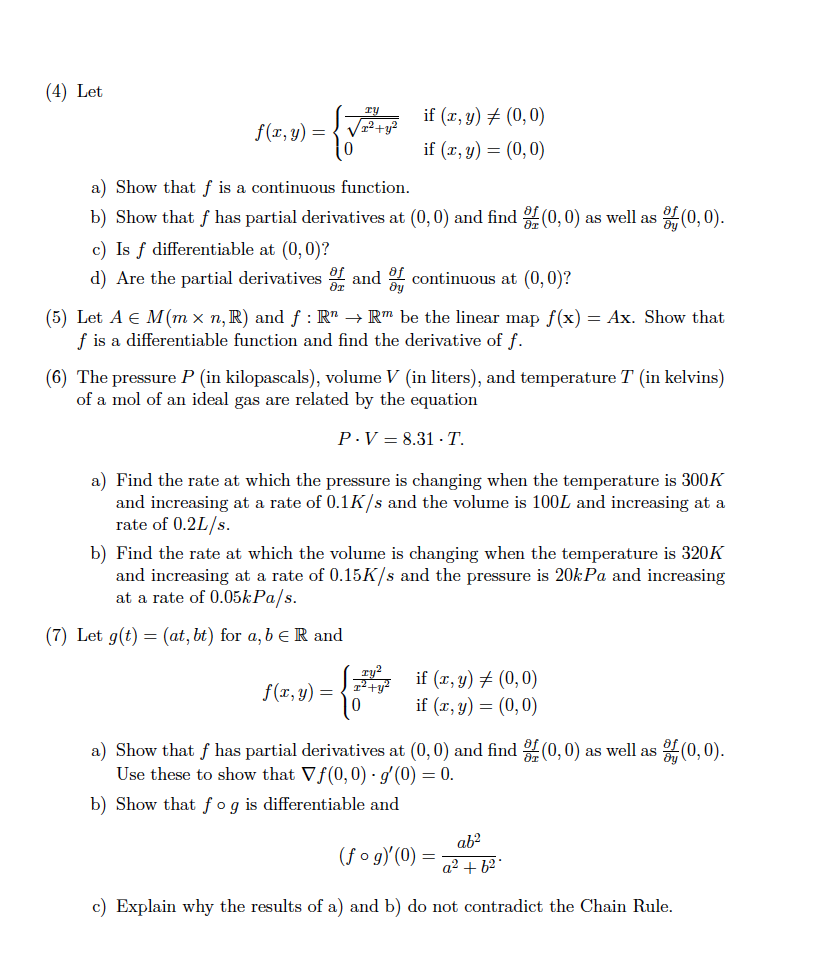Anyone can solve these questions? (4) Let if (z, y)メ(0,0) if (x, y) (0,0) f(z, y) / 0 a) Show that f is a continuous function b) Show that f has partial derivatives at (0,0) and find (0,0) as well as c) Is f differentiable at (0, 0)? d) Are the partial derivatives r tinuous at (0,0)? (0,0) (5) Let A E M(mx n,R) and f : RnRm be the linear map f(x)-Ax. Show that f is a differentiable function...